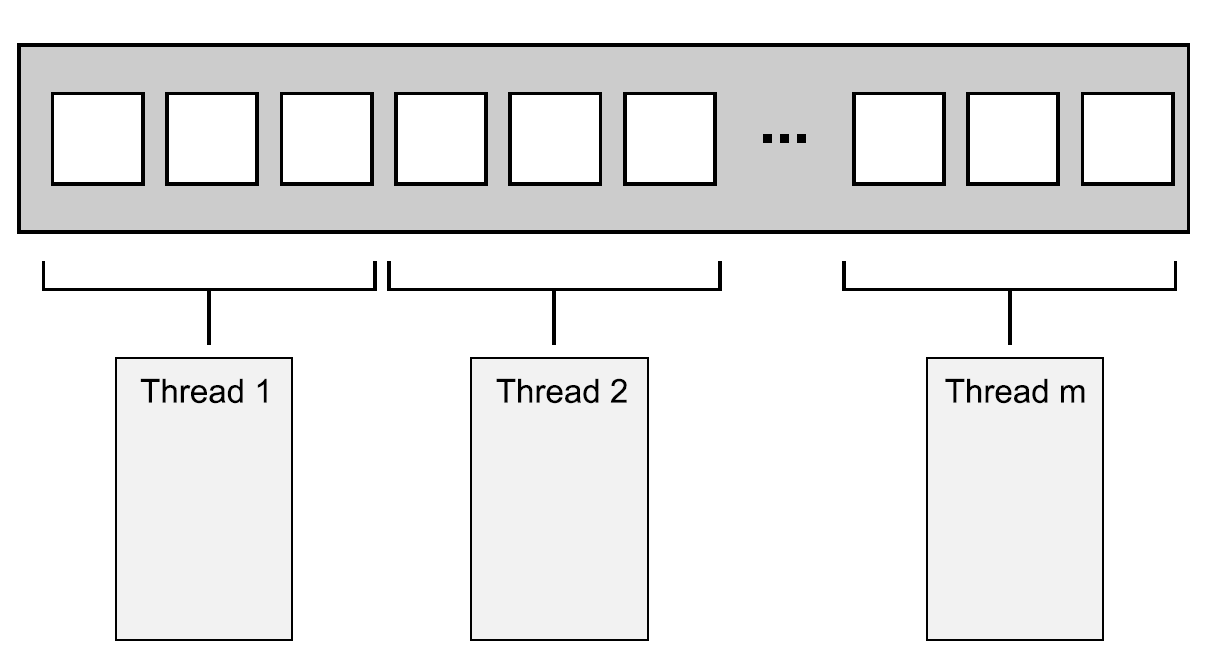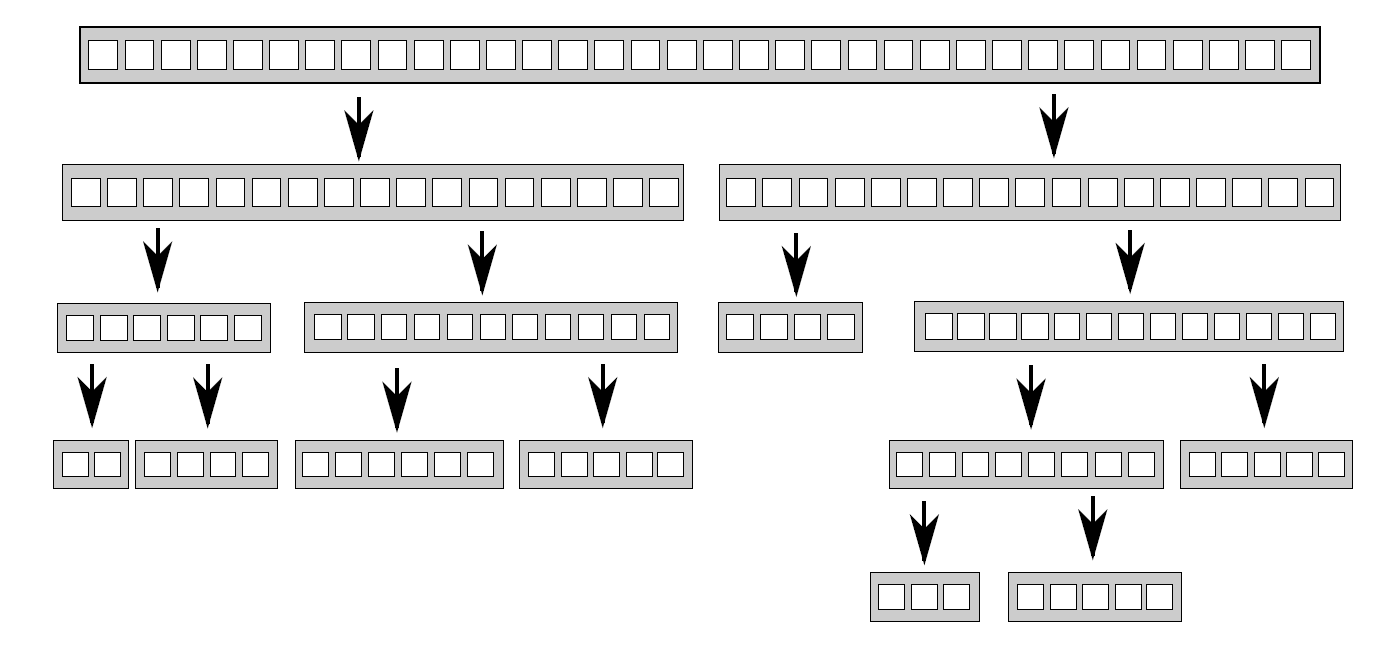8.1 线程间划分工作的技术

8.1.1 在线程处理前对数据进行划分8.1.2 递归划分template<typename T>
struct sorter  // 1
{
struct chunk_to_sort
{
std::list<T> data;
std::promise<std::list<T> > promise;
};

std::atomic<bool> end_of_data;

sorter():
end_of_data(false)
{}

~sorter()  // 4
{
end_of_data=true;  // 5

{
}
}

void try_sort_chunk()
{
boost::shared_ptr<chunk_to_sort > chunk=chunks.pop();  // 7
if(chunk)
{
sort_chunk(chunk);  // 8
}
}

std::list<T> do_sort(std::list<T>& chunk_data)  // 9
{
if(chunk_data.empty())
{
return chunk_data;
}

std::list<T> result;
result.splice(result.begin(),chunk_data,chunk_data.begin());
T const& partition_val=*result.begin();

typename std::list<T>::iterator divide_point=  // 10
std::partition(chunk_data.begin(),chunk_data.end(),
[&](T const& val){return val<partition_val;});

chunk_to_sort new_lower_chunk;
new_lower_chunk.data.splice(new_lower_chunk.data.end(),
chunk_data,chunk_data.begin(),
divide_point);

std::future<std::list<T> > new_lower=
new_lower_chunk.promise.get_future();
chunks.push(std::move(new_lower_chunk));  // 11
{
}

std::list<T> new_higher(do_sort(chunk_data));

result.splice(result.end(),new_higher);
while(new_lower.wait_for(std::chrono::seconds(0)) !=
{
try_sort_chunk();  // 14
}

result.splice(result.begin(),new_lower.get());
return result;
}

void sort_chunk(boost::shared_ptr<chunk_to_sort> const& chunk)
{
chunk->promise.set_value(do_sort(chunk->data));  // 15
}

{
while(!end_of_data)  // 16
{
try_sort_chunk();  // 17
}
}
};

template<typename T>
std::list<T> parallel_quick_sort(std::list<T> input)  // 19
{
if(input.empty())
{
return input;
}
sorter<T> s;

return s.do_sort(input);  // 20
}